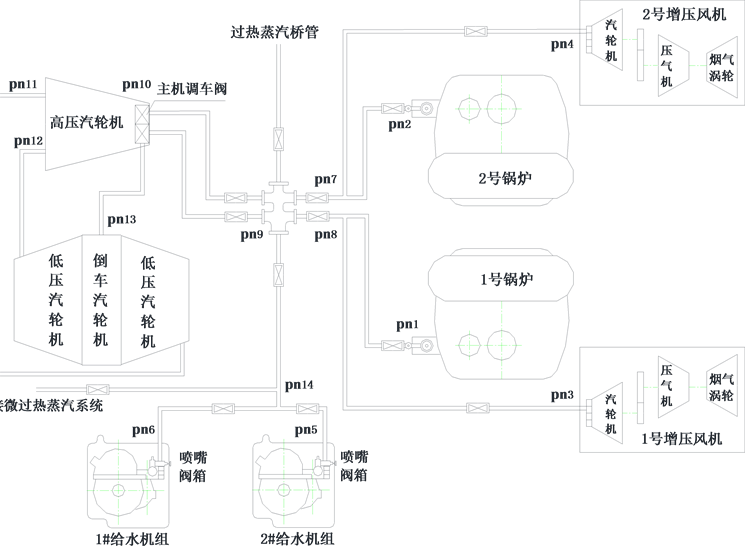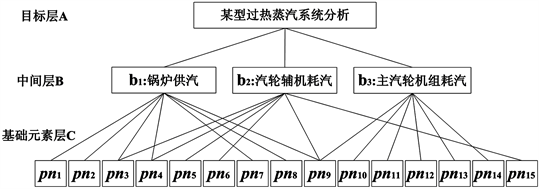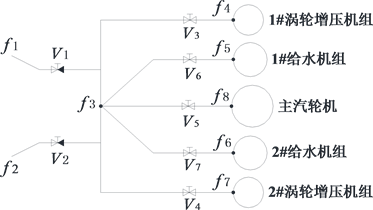﻿ 基于层次分析的热力系统模型简化方法研究 Study on Simplified Method of Thermal System Models Based on Hierarchical Analysis

Dynamical Systems and Control
Vol.07 No.01(2018), Article ID:23366,9 pages
10.12677/DSC.2018.71005

Study on Simplified Method of Thermal System Models Based on Hierarchical Analysis

Tao Hong1, Haibo Qin2

1Naval 91257 Force, Zhoushan Zhejiang

2College of Power Engineering, Naval University of Engineering, Wuhan HubeiReceived: Dec. 6th, 2017; accepted: Jan. 3rd, 2018; published: Jan. 10th, 2018ABSTRACT

The hierarchical analysis process used in system modeling and evaluation is applied to the model simplification of complex thermal systems in this paper. We grouped every system component elements in different levels through the analysis of system structure, thus formed an interconnected orderly hierarchy. And then the relative importance to study target of each element in system is determined and judged by numerical quantification, and a comparison matrix is structured based on the related information that provided by hierarchical analysis. Finally, according to the information contained in comparison matrix, the relative importance of each element in system is calculated and analysed, and the conditional simplification of thermal system models is realized by omitting some redundant or minor elements in the system.

Keywords:Model Simplified, Hierarchical Analysis, Thermal System

1海军91257部队，浙江 舟山

2海军工程大学电力工程学院，湖北 武汉1. 引言

2. 层次分析的理论基础

2.1. 基本思想

1) 系统分解

2) 重要性比较

3) 重要性计算

2.2. 数学基础

$A=\left(\begin{array}{cccc}{a}_{11}& {a}_{12}& \dots & {a}_{1n}\\ {a}_{21}& {a}_{22}& \dots & {a}_{2n}\\ ⋮& ⋮& \ddots & ⋮\\ {a}_{n1}& {a}_{n2}& \dots & {a}_{nn}\end{array}\right)=\left(\begin{array}{cccc}{\varphi }_{1}/{\varphi }_{1}& {\varphi }_{1}/{\varphi }_{2}& \dots & {\varphi }_{1}/{\varphi }_{n}\\ {\varphi }_{2}/{\varphi }_{1}& {\varphi }_{2}/{\varphi }_{2}& \dots & {\varphi }_{2}/{\varphi }_{n}\\ ⋮& ⋮& \ddots & ⋮\\ {\varphi }_{n}/{\varphi }_{1}& {\varphi }_{n}/{\varphi }_{2}& \dots & {\varphi }_{n}/{\varphi }_{n}\end{array}\right)$ (1)

$A\varphi =A{\left({\varphi }_{1},{\varphi }_{2},\dots ,{\varphi }_{n}\right)}^{\text{T}}=n\varphi$ (2)

$\underset{i=2}{\overset{n}{\sum }}{\lambda }_{i}=n-{\lambda }_{\mathrm{max}}<0$ (3)

${I}_{C}=\left({\lambda }_{\mathrm{max}}-n\right)/\left(n-1\right)$ (4)

Step 1：对于一个n阶比较矩阵，用随机方法构造m (m ≥ 200)个n阶单位矩阵。

Step 2：随机地用1~n标度中的n个整数及其倒数填满各单位矩阵的上三角部分，下三角的转置位置项取上三角对应位置随机数的倒数，构成m个随机样本矩阵。

Step 3：计算各随机样本矩阵的一致性指标IC，其平均值就是n阶比较矩阵的随机一致性指标IR

${R}_{C}={I}_{C}/{I}_{R}$ (5)

3. 层次分析的方法和步骤

3.1. 建立层次结构

3.2. 构造比较矩阵Table 1. The values of random coincidence indicator

${A}_{a-Y}=\left(\begin{array}{cccc}1& {a}_{12}& \dots & {a}_{1n}\\ {a}_{12}^{-1}& 1& \dots & {a}_{2n}\\ ⋮& ⋮& \ddots & ⋮\\ {a}_{n1}^{-1}& {a}_{n2}^{-1}& \dots & 1\end{array}\right)$ (6)

3.3. 同层元素排序

Step 1：计算矩阵每一行元素的乘积。

${\Theta }_{i}=\underset{j=1}{\overset{n}{\prod }}{a}_{ij},\text{\hspace{0.17em}}\text{\hspace{0.17em}}\left(i=1,2,\cdots ,n\right)$ (7)

Step 2：计算Θi的n次方根。

$\stackrel{¯}{{\Theta }_{i}}=\sqrt[n]{{\Theta }_{i}}$ (8)

Step 3：将 $\stackrel{¯}{\Theta }={\left(\stackrel{¯}{{\Theta }_{1}},\stackrel{¯}{{\Theta }_{2}},\cdots ,\stackrel{¯}{{\Theta }_{n}}\right)}^{\text{T}}$ 正规化，即为所求的最大特征向量 $\Phi ={\left({\Phi }_{1},{\Phi }_{2},\cdots ,{\Phi }_{n}\right)}^{\text{T}}$

${\varphi }_{i}=\stackrel{¯}{{\Theta }_{i}}/\underset{i=1}{\overset{n}{\sum }}\stackrel{¯}{{\Theta }_{i}},\text{\hspace{0.17em}}\text{\hspace{0.17em}}\left(i=1,2,\cdots ,n\right)$ (9)

Step 4：计算最大特征值。

${\lambda }_{\mathrm{max}}=\underset{i=1}{\overset{n}{\sum }}\frac{{\left(A\varphi \right)}_{i}}{n{\varphi }_{i}},\text{\hspace{0.17em}}\text{\hspace{0.17em}}\left(i=1,2,\cdots ,n\right)$ (10)Table 2. System resulting data of standard experiment

3.4. 层次总体排序

${\varphi }_{bi-X}=\underset{j=1}{\overset{n}{\sum }}{\lambda }_{aj}{\lambda }_{bi}^{j},\text{\hspace{0.17em}}\text{\hspace{0.17em}}\left(i=1,2,\cdots ,n\right)$ (11)

${I}_{C}^{*}=\underset{i=1}{\overset{n}{\sum }}{\lambda }_{ai}{I}_{Ci},\text{\hspace{0.17em}}\text{\hspace{0.17em}}{I}_{R}^{*}=\underset{i=1}{\overset{n}{\sum }}{\lambda }_{ai}{I}_{Ri}$ (12)

${R}_{C}^{*}={I}_{C}^{*}/{I}_{R}^{*}$ (13)

4. 应用实例

4.1. 对象说明

4.2. 分析与简化结果

$\begin{array}{l}{A}_{a-B}=\left(\begin{array}{ccc}1& 3& 5\\ 1/3& 1& 1\\ 1/5& 1& 1\end{array}\right),\text{\hspace{0.17em}}\text{\hspace{0.17em}}{A}_{{b}_{1}-C}=\left(\begin{array}{ccccccc}1& 1& 3& 3& 7& 7& 3\\ 1& 1& 3& 3& 7& 7& 3\\ 1/3& 1/3& 1& 1& 5& 5& 1/3\\ 1/3& 1/3& 1& 1& 5& 5& 1/3\\ 1/7& 1/7& 1/5& 1/5& 1& 1& 1/3\\ 1/7& 1/7& 1/5& 1/5& 1& 1& 1/3\\ 1/3& 1/3& 3& 3& 3& 3& 1\end{array}\right)\\ {A}_{{b}_{2}-C}=\left(\begin{array}{cccccc}1& 1& 1& 1& 1/3& 3\\ 1& 1& 1& 1& 1/3& 3\\ 1& 1& 1& 1& 1/5& 3\\ 1& 1& 1& 1& 1/5& 3\\ 3& 3& 5& 5& 1& 5\\ 1/3& 1/3& 1/3& 1/3& 1/5& 1\end{array}\right),\text{\hspace{0.17em}}\text{\hspace{0.17em}}{A}_{{b}_{3}-C}=\left(\begin{array}{ccccc}1& 5& 7& 7& 7\\ 1/5& 1& 7& 7& 7\\ 1/7& 1/7& 1& 1& 1\\ 1/7& 1/7& 1& 1& 1\\ 1/7& 1/7& 1& 1& 1\end{array}\right)\end{array}$ (14)Figure 1. Sketch of a certain marine superheated steam systemFigure 2. Hiberarchy of a certain type marine superheated steamTable 3. The maximal eigenvalue and eigenvector of relative matrix shown in Formula (14)Table 4. The total weightiness sort of press-nodes for a certain marine superheated steam system

5. 结论Figure 3. Topology structural map for superheated steam system after reduction

Study on Simplified Method of Thermal System Models Based on Hierarchical Analysis[J]. 动力系统与控制, 2018, 07(01): 52-60. http://dx.doi.org/10.12677/DSC.2018.71005

1. 1. 倪何. 复杂热力系统建模与技术状态评估[M]. 长沙: 国防科技大学出版社, 2016.

2. 2. 吕崇德, 任挺进, 姜学智, 等. 大型火电机组系统仿真与建模[M]. 北京: 清华大学出版社, 2002.

3. 3. 张荷芳, 贺为婷, 倪原. 大系统的一种模型降阶方法[J]. 西安工业学院学报, 2000, 20(3): 177-181.

4. 4. 孙世霞. 复杂大系统建模与仿真的可信性评估研究[D]: [博士学位论文]. 长沙: 国防科技大学, 2005.

5. 5. 李宁, 王李管, 贾明滔, 等. 基于层次分析法和证据理论的矿山井下六大系统安全评价[J]. 中南大学学报(自然科学版), 2014, 45(1): 287-292.

6. 6. 王新华, 李堂军, 丁黎黎. 复杂大系统评价理论与技术[M]. 济南: 山东大学出版社, 2010.